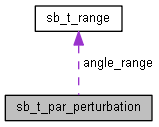SB SDK 1.11
sb_t_par_perturbation Struct Reference

Describes the perturbation of a sample. More...

`#include <sb.h>`

Collaboration diagram for sb_t_par_perturbation:[legend]

## Data Fields

int angle
Rotation angle, in degrees, of the sample. 1th operation. More...

int flip_horizontal
Flip around y-axis. 2th operation. More...

int flip_vertical
Flip around x-axis. 3th operation. More...

sb_t_range angle_range
Angular range, in degrees, for random rotation. More...

int num_synthetic_samples
Number of synthetic sample to generated with a random angle in the range sb_t_par_perturbation::angle . More...

## Detailed Description

Describes the perturbation of a sample.

Shallow Learning Perturbations

Definition at line 9291 of file sb.h.

## ◆ angle

 int sb_t_par_perturbation::angle

Rotation angle, in degrees, of the sample. 1th operation.

The range of possible values is [-180;+180] degrees.

Definition at line 9297 of file sb.h.

## ◆ angle_range

 sb_t_range sb_t_par_perturbation::angle_range

Angular range, in degrees, for random rotation.

sb_t_par_perturbation::num_synthetic_samples is the number of synthetic sample to generated at a random angle.
Minimum angle must be less than or equal that maximum angle.
The range of values for both the minimum angle and the maximum angle is [-180; 180] degrees.

Definition at line 9321 of file sb.h.

## ◆ flip_horizontal

 int sb_t_par_perturbation::flip_horizontal

Flip around y-axis. 2th operation.

• 0 = disabled
• 1 = enabled

Definition at line 9305 of file sb.h.

## ◆ flip_vertical

 int sb_t_par_perturbation::flip_vertical

Flip around x-axis. 3th operation.

• 0 = disabled
• 1 = enabled

Definition at line 9313 of file sb.h.

## ◆ num_synthetic_samples

 int sb_t_par_perturbation::num_synthetic_samples

Number of synthetic sample to generated with a random angle in the range sb_t_par_perturbation::angle .

If 0 no synthetic samples are generated.
The range of possible values is [0;32]

Definition at line 9328 of file sb.h.

The documentation for this struct was generated from the following file: